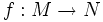# Critical point set is closed

Let$f:M \to N$ be a smooth map of differential manifolds. Then, the set of critical points of$f$ is a closed subset of$M$; equivalently, the set of regular points of$f$ is an open subset of$M$.